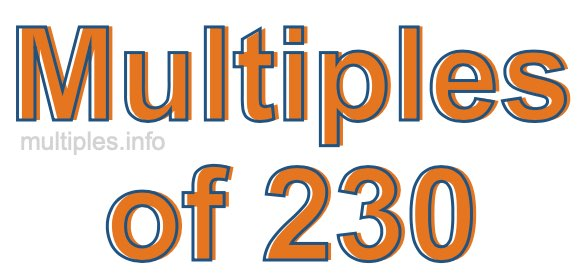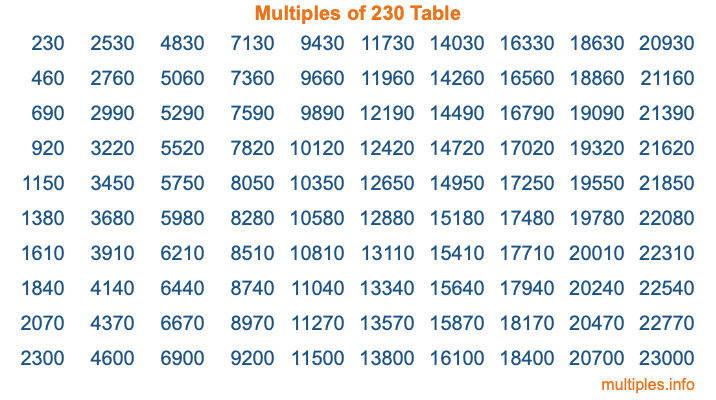Multiples of 230Welcome to the Multiples of 230 page. Here we will first teach you everything you will ever need to know about the multiples of 230, and then give you a study guide summary of everything we taught you to make sure you remember it all. Use this page to look up facts and learn information about the multiples of 230. This page will make you a multiples of two hundred thirty expert!

Definition of Multiples of 230
Multiples of 230 are all the numbers that when divided by 230 equal an integer. Each of the multiples of 230 are called a multiple. A multiple of 230 is created by multiplying 230 by an integer.

Therefore, to create a list of multiples of 230, you start with 1 multiplied by 230, then 2 multiplied by 230, then 3 multiplied by 230, and so on for as long as you want. Thus, the list of the first five multiples of 230 is 230, 460, 690, 920, and 1150. To see a larger list of multiples of 230, see the printable image of Multiples of 230 further down on this page. We also have a category where you can choose any nth multiple of 230.

Multiples of 230 Checker
The Multiples of 230 Checker below checks to see if any number of your choice is a multiple of 230. In other words, it checks to see if there is any number (integer) that when multiplied by 230 will equal your number. To do that, we divide your number by 230. If the the quotient is an integer, then your number is a multiple of 230.

Is  a multiple of 230?

Least Common Multiple of 230 and ...
A Least Common Multiple (LCM) is the lowest multiple that two or more numbers have in common. This is also called the smallest common multiple or lowest common multiple and is useful to know when you are adding our subtracting fractions. Enter one or more numbers below (230 is already entered) to find the LCM.

Check out our LCM Calculator if you need more details about the Least Common Multiple or if you need the LCM for different numbers for adding and subtraction fractions.

nth Multiple of 230
As we stated above, 230 is the first multiple of 230, 460 is the second multiple of 230, 690 is the third multiple of 230, and so on. Enter a number below to find the nth multiple of 230.

th multiple of 230

Multiples of 230 vs Factors of 230
230 is a multiple of 230 and a factor of 230, but that is where the similarities end. All postive multiples of 230 are 230 or greater than 230. All positive factors of 230 are 230 or less than 230.

Below is the beginning list of multiples of 230 and the factors of 230 so you can compare:

Multiples of 230: 230, 460, 690, 920, 1150, etc.

Factors of 230: 1, 2, 5, 10, 23, 46, 115, 230

As you can see, the multiples of 230 are all the numbers that you can divide by 230 to get a whole number. The factors of 230, on the other hand, are all the whole numbers that you can multiply by another whole number to get 230.

It's also interesting to note that if a number (x) is a factor of 230, then 230 will also be a multiple of that number (x).

Multiples of 230 vs Divisors of 230
The divisors of 230 are all the integers that 230 can be divided by evenly. Below is a list of the divisors of 230.

Divisors of 230: 1, 2, 5, 10, 23, 46, 115, 230

The interesting thing to note here is that if you take any multiple of 230 and divide it by a divisor of 230, you will see that the quotient is an integer.

Multiples of 230 Table
Below is an image of the first 100 multiples of 230 in a table. The table is in chronological order, column by column. The first column has the first ten multiples of 230, the second column has the next ten multiples of 230, and so on.The Multiples of 230 Table is also referred to as the 230 Times Table or Times Table of 230. You are welcome to print out our table for your studies.

Negative Multiples of 230
Although not often discussed or needed in math, it is worth mentioning that you can make a list of negative multiples of 230 by multiplying 230 by -1, then by -2, then by -3, and so on, to get the following list of negative multiples of 230:

-230, -460, -690, -920, -1150, etc.

Multiples of 230 Summary
Below is a summary of important Multiples of 230 facts that we have discussed on this page. To retain the knowledge on this page, we recommend that you read through the summary and explain to yourself or a study partner why they hold true.

There are an infinite number of multiples of 230.

A multiple of 230 divided by 230 will equal a whole number.

230 divided by a factor of 230 equals a divisor of 230.

The nth multiple of 230 is n times 230.

The largest factor of 230 is equal to the first positive multiple of 230.

230 is a multiple of every factor of 230.

230 is a multiple of 230.

A multiple of 230 divided by a divisor of 230 equals an integer.

230 divided by a divisor of 230 equals a factor of 230.

Any integer times 230 will equal a multiple of 230.

Multiples of a Number
Here you can get the multiples of another number, all with the same attention to detail as we did for multiples of 230 on this page.

Multiples of
Multiples of 231
Did you find our page about multiples of two hundred thirty educational? Do you want more knowledge? Check out the multiples of the next number on our list!